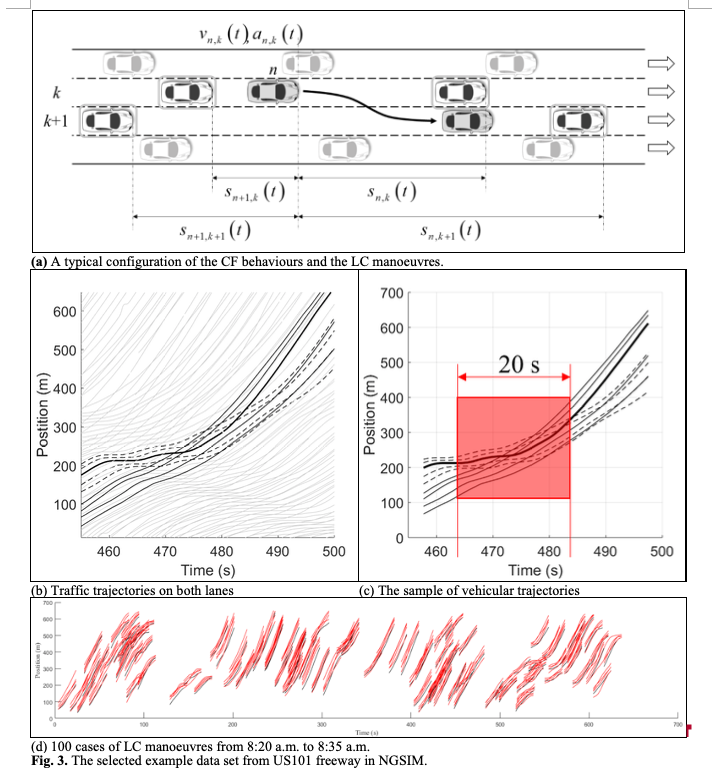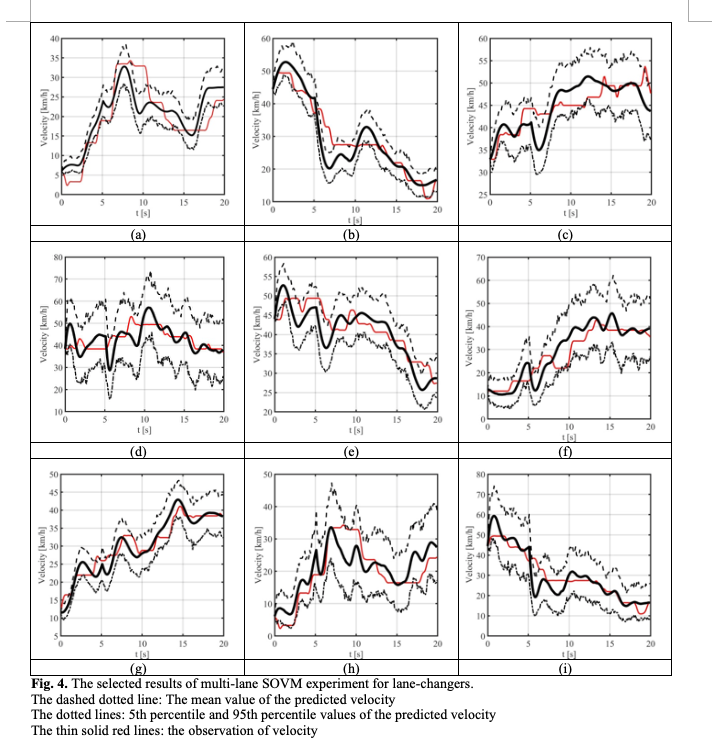A.I. For Car-Following Models

We develop an integrated multi-lane stochastic continuous car-following model where a deep learning architecture is used to estimate a probability of lane-changing (LC) manoeuvres. The objective of this study is to establish a coupled stochastic continuous multi-lane car-following model using Langevin equations to cope with probabilistic characteristics of LC manoeuvres. In particular, a stochastic volatility, derived from LC manoeuvres is introduced in a multi-lane stochastic optimal velocity model (SOVM). In additions, Convolutional Neural Network (CNN) is applied to estimate a probability of LC manoeuvres in the integrated multi-lane car-following model. Furthermore, imaged second-based trajectories of the lane-changer and surrounding vehicles are used to identify whether LC manoeuvres occur by using the CNN. A typical schematic configuration of the CF behaviours and the LC manoeuvres is illustrated in Figure 3 to facilitate an effective understanding.We illustrate a fluctuation in velocity of 9 representative lane-changers for each 10s before and after each LC manoeuvres in Figure 4. The highlighted solid lines denote mean value of predicted velocity for each case with the dashed dotted lines and the dotted lines as 5th percentile and 95th percentile, respectively. In additions, the thin solid red lines show the observation of velocity. The average driving velocity is not very high in this section because the time-period for the validation is the typical morning peak-hour. For all cases, the proposed model well estimates trajectories of the lane-changers with respect to fluctuation in the observed velocity of the corresponding lane-changers.

Finally, the proposed method is validated using a real-world high-resolution vehicle trajectory dataset. The results indicate that the prediction of the integrated SOVM is almost identical to the observed trajectories of the lane-changers and the following vehicles in the initial and the target lane. It has been found that the proposed multi-lane SOVM can tackle the unpredictable fluctuations in the velocity of the vehicles in the acceleration/deceleration zone.Reference:

• Seunghyeon Lee, Dong Ngoduy, Mehdi Keyvan-Ekbatani, 2019. Integrated deep learning and stochastic car-following model for traffic dynamics on multi-lane freeways. Transportation Research Part C: Emerging Technologies, in press.